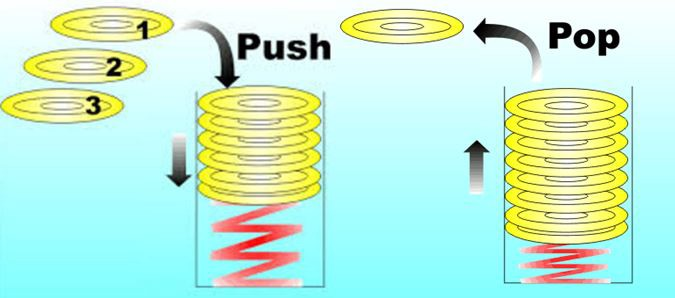## 汇编相关知识介绍

### 理解虚拟机和栈

``````contract Assembler {
function do_something_cpu() public {
assembly {
// start writing evm assembler language
}
}
}``````EVM是一个基于栈的虚拟机（stack machine），栈这种数据结构只允许两个操作：压入（PUSH）或弹出（POP）数据。 最后压入的数据位于栈顶，因此将被第一个弹出，这被称为后进先出 （LIFO：Last In, First Out）：

stack machine 也是一种处理器，其中所有操作数都存储在栈中。 它仍然具有PC（程序计数器）和SP（堆栈指针）的存储器和寄存器，但是所有内容都存储在栈中。

### 操作码基础

``````a + b      // 标准表示法Infix

## 更少的Gas消耗

``````function addAssembly(uint x, uint y) public pure returns (uint) {
assembly {
mstore(0x0, result)
return(0x0, 32)
}
}

function addSolidity(uint x, uint y) public pure returns (uint) {
return x + y;
}``````## Solidity 中两种类型的汇编

• 内联汇编（Inline Assembly )：可以在内部Solidity源代码中使用。
• 独立汇编（Standalone Assembly：可以使用，无需Solidity。

## 基本汇编语法

### 引入汇编

``````assembly {
// some assembly code here
}``````

`assembly`块内的代码开发语言被称为Yul，为了简化我们称其为 汇编、汇编代码或EVM汇编。

Solidity 文档有这样的解释： 不同的内联汇编块不共享任何名称空间，即不可能调用Yul函数或访问在其他内联汇编块中定义的Yul变量。

``````assembly {
let x := 2
}

assembly {
let y := x          // Error
}
// let y := x
// ^``````

### 简单汇编示例

``````function addition(uint x, uint y) public pure returns (uint) {
assembly {
let result := add(x, y)   // x + y
mstore(0x0, result)       // 在内存中保存结果
return(0x0, 32)           // 从内存中返回32字节
}
}
``````

``````function addition(uint x, uint y) public pure returns (uint) {
assembly {
// 创建一个新的变量result
//     -> 将计算结果赋值给变量result
let result := add(x, y)   // x + y

// 使用mstore操作码
//     -> 将result变量的值存入内存
//     -> 指定内存地址 0x0
mstore(0x0, result)       // 将结果存入内存

// 从内存地址0x返回32字节
return(0x0, 32)
}
}``````

### Solidity汇编中的变量定义与赋值

``````assembly {
let x := 2
}``````

``````assembly {
let x           // 自动初始化为 x = 0
x := 5          // x 现在的值是5
}
``````

``````assembly {
let x := 7
let z := add(keccak256(0x0, 0x20), div(slength, 32))
let n  // 自动初始化为 n = 0
}``````

#### 汇编中let指令的运行机制

• 创建一个新的堆栈槽位
• 为变量保留该槽位
• 当到达代码块结束时自动销毁该槽位

### 汇编的注释

``````assembly {
// 单行注释

/*
多
行
注释
*/
}``````

## 汇编中的字面量

``````assembly {
let a := 0x123             // 16进制
let b := 42                // 10进制
let c := "hello world"     // 字符串

let d := "very long string more than 32 bytes" // 超长字符串，出错！
}

// TypeError: String literal too long (35 &lt; 32)
// let d := "really long string more than 32 bytes"
// ``````

### 汇编中的块和作用域

``````assembly {
let x := 3          // x在各处可见

// Scope 1
{
let y := x     // ok
}  // 到此处会销毁y

// Scope 2
{
let z := y     // Error
} // 到此处会销毁z
}

// let z := y
// ^
``````

## 在Solidity汇编中访问变量

### 局部变量

``````function assembly_local_var_access() public pure {
uint b = 5;

assembly {                // defined inside  an assembly block
let y := 10
}

assembly {               // defined outside an assembly block
let y := mul(x, b)
}
}``````

## 汇编中的循环

### For循环

``````function for_loop_solidity(uint n, uint value) public pure returns(uint) {

for ( uint i = 0; i &lt; n; i++ ) {
value = 2 * value;
}
return value;
}``````

``````function for_loop_assembly(uint n, uint value) public pure returns (uint) {

assembly {

for { let i := 0 } lt(i, n) { i := add(i, 1) } {
value := mul(2, value)
}

mstore(0x0, value)
return(0x0, 32)

}

} ``````

• 初始化：`let i := 0`
• 执行条件：`lt(i, n)` ，必须是函数风格表达式
• 迭代后续步骤：`add(i, 1)`

### 汇编中的 while循环

``````assembly {
let x := 0
let i := 0
for { } lt(i, 0x100) { } {     // 等价 while(i &lt; 0x100)
}
}``````

## 在Solidity汇编的判断语句

### 使用If

Solidity内联汇编支持使用`if`语句来设置代码执行的条件，但是没有其他语言中的`else`部分。

``````assembly {
if slt(x, 0) { x := sub(0, x) }  // Ok

if eq(value, 0) revert(0, 0)    // Error, 需要大括号
}``````

if语句强制要求代码块使用大括号，即使需要保护的代码只有一行， 也需要使用大括号。这和Solidity 有点不同。

``````function calculate_delivery_charges(uint ether_sent) public pure returns (uint) {

// free delivery on orders less than 0.25 ethers

if ( ether_sent &lt; 0.25 ether) revert();

// more code...

}``````

### 使用switch语句

EVM汇编中也有`switch`语句，它将一个表达式的值于多个常量进行对比，并选择相应的代码分支来执行。`switch`语句支持 一个默认分支`default`，当表达式的值不匹配任何其他分支条件时，将执行默认分支的代码。

``````assembly {
let x := 0
case 0 {
}
default {
}
sstore(0, div(x, 2))
}``````

`switch`语句在语法上有一些限制：

• 分支列表不需要大括号，但是分支的代码块需要大括号；
• 所有的分支条件值必须：1）具有相同的类型 2）具有不同的值
• 如果分支条件已经涵盖所有可能的值，那么不允许再出现default条件
``````assembly {

let x := 34

switch lt(x, 30)
case true {
// do something
}
case false {
// do something els
}
default {
// 不允许
}

}``````

1. Solidity 支持 `if { ... } else { ... }` 语句, `while`, `do``for` 循环, 但是没有 `switch` 语句。
2. 当比较的值与一个 `case` 匹配时，控制流将停止。它不会从一个`case`延续到下一个`case`

• 作为非常基本版本的"if/else"
• 作为 if 语句的扩展版本

## Solidity汇编的函数

``````assembly {

function allocate(length) -> pos {
}
let free_memory_pointer := allocate(64)
}``````

1. 从栈顶提取参数
2. 将结果压入栈

### 函数定义

``````assembly {

function my_assembly_function(param1, param2) {
// assembly code here

}
}``````

### 函数返回值

``````assembly {

function my_assembly_function(param1, param2) -> my_result {

// param2 - (4 * param1)
my_result := sub(param2, mul(4, param1))

}
let some_value = my_assembly_function(4, 9)  // 4 - (9 * 4) = 32
}``````

### 退出函数

`leave` 关键字可以放置在汇编函数体的任意位置，以停止其执行流并退出它。它的工作原理与空返回语句完全相同，有一个例外：函数将返回上次复制的变量给返回变量。

## 操作码(Opcodes)和汇编

### 操作码简介

EVM 操作码可以分为以下几类：

• 算数和比较操作
• 位操作
• 密码学计算，目前仅包含`keccak256`
• 环境操作码，主要指与区块链相关的全局信息，例如：`blockhash``coinbase`
• 存储、内存和栈操作
• 交易与合约调用操作
• 停机操作
• 日志操作

### 有哪些操作码

• 操作码始终从堆栈顶部获取参数（在括号中给出）。

• 标有 `-`（第二列）的操作码不会将项推送到栈上。这些操作码的大多数是返回内存中的值。

• 所有其他操作码会将项（其"返回"值）压入到堆栈上。

• 标有`F``H``B``C`的操作码，表示从相应的版本：Frontier、Homestead、Byzantium和Constantinople（君士坦丁堡）加入。

译者注： 以太坊经历了哪些升级版本，可以查看：以太坊发展简史

• `mem[a...b)` 表示从位置 a 开始到（但不包括位置 b）开始的内存字节。

• `storage[p]` 表示位置 p 处的存储内容。

## 高级的汇编概念

### 多个赋值

``````assembly {

function f() -> a, b {}
let c, d := f()

}``````

## Solidity中的独立汇编（Standalone Assembly）

### 直接的栈控制

• `dup1``dup16`的du``p指令允许在堆栈上复制值。
• `swap`指令（从swap1到swap16）允许交换堆栈上的值。

## Solidity中的函数式风格汇编

``````// Functional style

``````// Non-Functional style

• 函数式： 更容易了解哪个操作数（operand）作用于哪个操作码（opcode）。
• 非函数式：更容易看到这些值最终在堆栈中的位置。

``````mstore(0x80, add(mload(0x80), 3))          // Functional style

// Layout of the stack after each instructions
|_0x80| > |__5__|             |_0x80|
|_____| > |__3__| > |__3__| > |__3__| > |__8__| > |__8__| > |_____|``````

## 编写汇编的缺点

Solidity文档指出以下内容：

## 汇编中都是uint

EVM汇编中的算术运算忽略了某些类型可以小于256位的（例如：uint64）。

• 发表于 2020-02-23 20:58
• 阅读 ( 6033 )
• 学分 ( 192 )
• 分类：Solidity